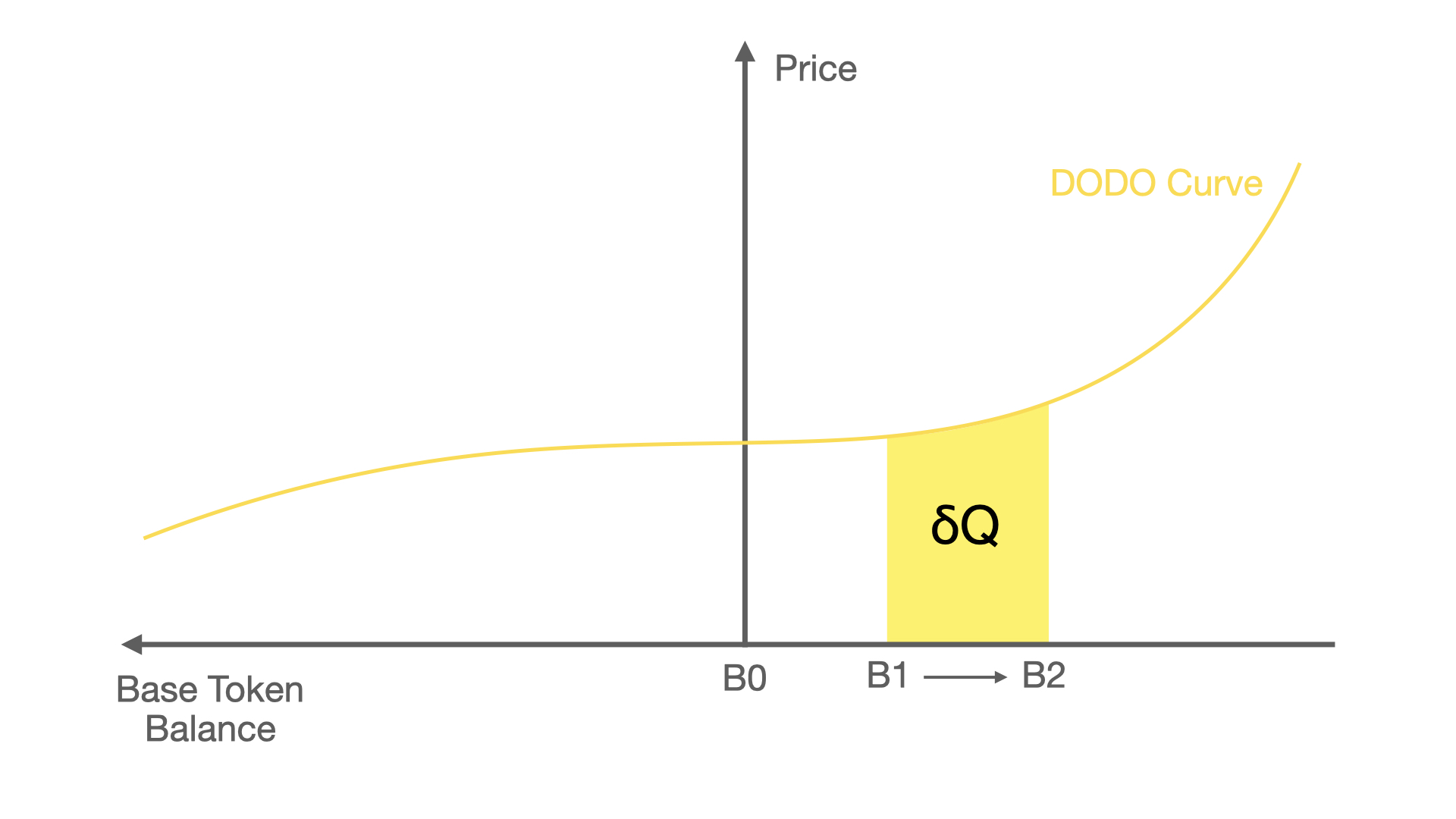# PMM 核心#

PMM 本质上是利用智能合约求解一个积分和两个二次方程的算法。

## 价格曲线积分#$\Delta Q =\int^{B_2}_{B_1}P_{margin}dB$

$= \int^{B_2}_{B_1}(1-k)i+i(B_0/B)^2kdB$

$= i(B_2-B_1)*(1-k+k\frac{B_0^2}{B_1B_2})$

## 求解交易的二次方程#

$\Delta B = \frac{1}{i}(Q_2-Q_1)*(1-k+k\frac{Q_0^2}{Q_1Q_2})$

$\Delta B, Q_0, Q_1$ 已知，我们可以通过推导二次方程得出 $Q_2$ ，把二次方程做个变形可以得到：

$(1-k)Q_2^2+(\frac{kQ_0^2}{Q_1}-Q_1+kQ_1-i\Delta B)Q_2-kQ_0^2=0$

$let \ a=1-k, \ b=\frac{kQ_0^2}{Q_1}-Q_1+kQ_1-i\Delta B, \ c=-kQ_0^2$

$Q_2=\frac{-b+\sqrt{b^2-4ac}}{2a}$

• $\Delta B>0$ 时, $Q_2>Q_1$; 交易者想买 base token 时，需要支付 $Q_2-Q_1$
• $\Delta B<0$ 时, $Q_2; 交易者想出售 base token 时，会收到 $Q_1-Q_2$
• $\Delta B=0$ 时, $Q_2=Q_1$.

## 求解回归目标的二次方程#

Given $\Delta Q = i(B_2-B_1)*(1-k+k\frac{B_0^2}{B_1B_2})$

$\frac{k}{B_1}B_0^2+(1-2k)B_0-[(1-k)B_1+\frac{\Delta Q}{i}] = 0$

$B_0=B_1+B_1\frac{\sqrt{1+\frac{4k\Delta Q}{B_1 i}}-1}{2k}$

$Q_0=Q_1+Q_1*\frac{\sqrt{1+\frac{4k\Delta B i}{Q_1}}-1}{2k}$

# 其他#

## 充值#

注意：充值奖励并不是无风险的套利交易机会。

## 提取#

PMM 算法要求在这种情况下，提取需要支付一定的手续费。手续费等于这笔引起的做市商的亏损总和。这笔手续费将会被分配给还未提取的做市商。